# PSEB 12th Class Physics Solutions Chapter 9 Ray Optics and Optical Instruments

Punjab State Board PSEB 12th Class Physics Book Solutions Chapter 9 Ray Optics and Optical Instruments Textbook Exercise Questions and Answers.

## PSEB Solutions for Class 12 Physics Chapter 9 Ray Optics and Optical Instruments

PSEB 12th Class Physics Guide Ray Optics and Optical Instruments Textbook Questions and Answers

Question 1.
A small candle, 2.5 cm in size is placed at 27 cm in front of a concave mirror of radius of curvature 36 cm. At what distance from the mirror should a screen be placed in order to obtain a sharp image? Describe the nature and size of the image. If the candle is moved closer to the mirror, how would the screen have to be moved?
Size of the candle, h = 2.5 cm
Image size = h’
Object distance, u = -27 cm
Radius of curvature of the concave mirror, R = -36 cm
Focal length of the concave mirror, f = $$\frac{R}{2}=\frac{-36}{2}$$ = -18 cm
Image distance = v

The image distance can be obtained using the mirror formula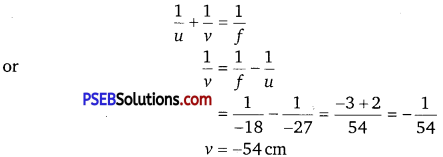The negative sign shows that the image is formed in front of the mirror i.e., on the side of the object itself. Thus the screen must be placed at a distance of 54 cm in front of the mirror.
The magnification of the image is given as

The height of the candle’s image is 5 cm. The negative sign indicates that the image is inverted and virtual.
If the candle is moved closer to the mirror, then the screen will have to be moved away from the mirror in order to obtain the image.

Question 2.
A 4.5 cm needle is placed 12 cm away from a convex mirror of focal length 15 cm. Give the location of the image and the magnification. Describe what happens as the needle is moved farther from the mirror.
Given u = -12 cm, f = +15 cm. (convex mirror)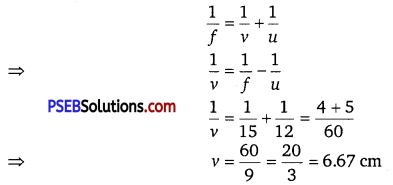That is image is formed at a distance of 6.67 cm behind the mirror.
Magnification m = $$-\frac{v}{u}=-\frac{\frac{20}{3}}{-12}$$ = $$\frac{5}{9}$$
Size of image I = mO = $$\frac{5}{9}$$ x 4.5 = 2.5 cm
The image is erect, virtual and has a size 2.5 cm.

Its position is 6.67 cm behind the mirror when needle is moved farther, the image moves towards the focus and its size goes on decreasing.

Question 3.
A tank is filled with water to a height of 12.5 cm. The apparent depth of a needle lying at the bottom of the tank is measured by a microscope to be 9.4 cm. What is the refractive index of water? If water is replaced by a liquid of refractive index 1.63 up to the same height, by what distance would the microscope have to be moved to focus on the needle again?
Case I:
When tank is filled with water Actual depth of the needle in water, h1 = 12.5cm
Apparent depth of the needle in water, h2 =9.4cm
Refractive index of water = μ
The value μ can be obtained as follows
μ = $$\frac{\text { Actual depth }}{\text { Apparent depth }}$$
= $$\frac{h_{1}}{h_{2}}=\frac{12.5}{9.4}$$ ≈ 1.33
Hence, the refractive index of water is about 1.33

Case II: When tank is filled with liquid
Water is replaced by a liquid of refractive index, μ’ = 1.63
The actual depth of the needle remains the same, but its apparent depth changes.
Let y be the new apparent depth of the needle. Hence, we can write the relation
μ’ = $$\frac{h_{1}}{y}$$
y = $$\frac{h_{1}}{\mu^{\prime}}=\frac{12.5}{1.63}$$ = 7.67 cm
Hence, the new apparent depth of the needle is 7.67cm. It is less than h2 Therefore, to focus the needle again, the microscope should be moved up. Distance by which the microscope should be moved up =9.4-7.67 = 1.73 cm.

Question 4.
Figures 9.34 (a) and (b) show refraction of a ray in air incident at 60° with the normal to a glass air and water-air interface, respectively. Predict the angle of refraction in glass when the angle of incidence in water is 45° with the normal to a water-glass interface [Fig. 9.34 (c)]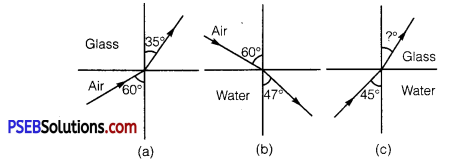As per the given figure, for the glass-air interface
Angle of incidence, i = 60°
Angle of refraction, r = 35°
The relative refractive index of glass with respect to air is given by Snell’s law as
aμg = $$\frac{\sin i}{\sin r}$$
= $$\frac{\sin 60^{\circ}}{\sin 35^{\circ}}=\frac{0.8660}{0.5736}$$ = 1.51 …………………….. (1)
As per the given figure, for the air-water interface
Angle of incidence, j = 600
Angle of refraction, r = 470
The relative refractive index of water with respect to air is given by Snell’s law as
wμw = $$\frac{\sin i}{\sin r}$$
= $$\frac{\sin 60^{\circ}}{\sin 47^{\circ}}=\frac{0.8660}{0.7314}$$ = 1.184 …………………………… (2)

Using equations (1) and (2), the relative refractive index of glass with respect to water can be obtained as
wμg = $$\frac{a_{g}}{a_{w_{w}}}$$
= $$\frac{1.51}{1.184}$$ = 1.275

The following figure shows the situation involving the glass-water interface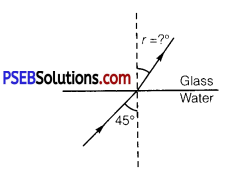Angle of incidence, i = 45
Angle of reflection = r
From Snell’s law, r can be calculated as, $$\frac{\sin i}{\sin r}$$ = wμg
$$\frac{\sin 45^{\circ}}{\sin r}$$ = 1.275
sin r = $$\frac{\frac{1}{\sqrt{2}}}{1.275}=\frac{0.707}{1.275}$$ = 0.5546
r = sin-1(0.5546) = 38.68°
Hence, the angle of refraction at the water-glass interface is 38.68°

Question 5.
A small bulb is placed at the bottom of a tank containirg water to a depth of 80 cm. What is the area of the surface of water through which light from the bulb can emerge out? Refractive index of water is 1.33 (Consider the bulb to be a point source.)
Actual depth of the bulb in water, d1 = 80 cm = 0.8 m
Refractive index of water, μ = 1.33
The given situation is shown in the following figure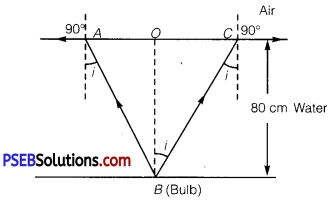where,
i = Angle of Incidence
r = Angle of Refraction = 90°
Since the bulb is a point source, the emergent light can be considered as a circle of radius,
R = $$\frac{A C}{2}$$ = AO = OC
Using Snell’s law, we can write the relation for the refractive index of water as
μ = $$\frac{\sin r}{\sin i}$$
1.33 = $$\frac{\sin 90^{\circ}}{\sin i}$$
i = sin-1$$\left(\frac{1}{1.33}\right)$$ = 48.75°

Using the given figure, we have the relation
tan i = $$\frac{O C}{O B}=\frac{R}{d_{1}}$$
∴R = tan 48.75° x 0.8 = 0.91 m
∴ Area of the surface of water = πR2
= π(0.91)2
= 2.61 m2
Hence, the area of the surface of water through which the light from the bulb can emerge is approximately 2.61 m2.

Question 6.
A prism is made of glass of unknown refractive index. A parallel beam of light is incident on a face of the prism. The angle of minimum deviation is measured to be 40°. What is the refractive index of the material of the prism? The refracting angle of the prism is 60°. If the prism is placed in water (refractive index 1.33), predict the new angle of minimum deviation of a parallel beam of light.
Angle of minimum deviation, δm = 40 °
Refracting angle of the prism, A = 60°
Refractive index of water, μ = 1.33
Let μ’ be the refractive index of the material of the prism.
The angle of deviation and refracting angle of the prism are related to refractive index (μ’) as
μ’ = $$\frac{\sin \left(\frac{A+\delta_{m}}{2}\right)}{\sin \left(\frac{A}{2}\right)}$$
= $$\frac{\sin \left(\frac{60^{\circ}+40^{\circ}}{2}\right)}{\sin \left(\frac{60^{\circ}}{2}\right)}=\frac{\sin 50^{\circ}}{\sin 30^{\circ}}=\frac{0.766}{0.5}$$
= 1.532
Hence, the refractive index of the material of the prism is 1.532.
Since the prism is placed in water, let 8 ^ be the new angle of minimum deviation for the same prism.

The refractive index of glass with respect to water is given by the relation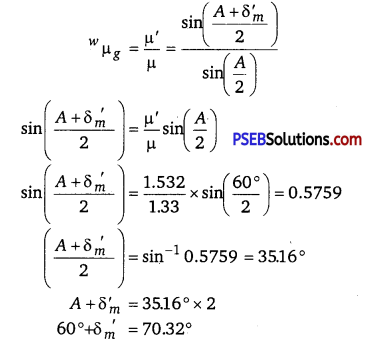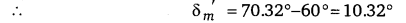Hence, the new minimum angle of deviation is 10.32°.

Question 7.
Double-convex lenses are to be manufactured from a glass of refractive index 1.55, with both faces of the same radius of curvature. What is the radius of curvature required if the focal length is to be 20 cm?
Lens maker formula is
$$\frac{1}{f}=(n-1)\left(\frac{1}{R_{1}}-\frac{1}{R_{2}}\right)$$ …………………………………… (1)
If R is radius of curvature of double convex lens, then,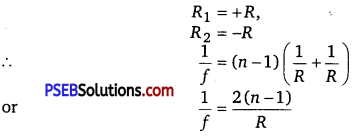∴ R = 2(n-1)f
Here, n =1.55, f = +20 cm
∴ R = 2 (1.55 -1) x 20 = 22 cm

Question 8.
A beam of light converges at a point P. Now a lens is placed in the path of the convergent beam 12 cm from P. At what point does the beam converge if the lens is (a) a convex lens of focal length 20 cm, and (b) a concave lens of focal length 16 cm?
In the given situation, the object is virtual and the image formed is real.
Object distance, u = +12cm
(a) Focal length of the convex lens, f = 20 cm
Image distance = v
According to the lens formula, we have the relation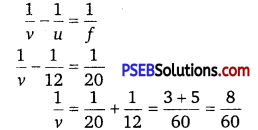∴ v = $$\frac{60}{8}$$ = 7.5cm
Hence, the image is formed 7.5cm away from the lens, toward its right.

(b) Focal length of the concave lens, f = -16 cm
Image distance = v
According to the lens formula, we have the relation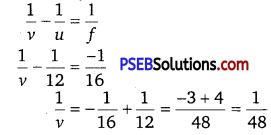∴ v = 48 cm
Hence, the image is formed 48 cm away from the lens, toward its right.

Question 9.
An object of size 3.0 cm is placed 14 cm in front of a concave lens of focal length 21 cm. Describe the image produced by the lens. What happens if the object is moved further away from the lens?
Size of object O = 3.0 cm
u = -14 cm, f = -21 cm (concave lens)
∴ Formula $$\frac{1}{f}=\frac{1}{v}-\frac{1}{u}$$
⇒ $$\frac{1}{v}=\frac{1}{f}+\frac{1}{u}$$
or $$\frac{1}{v}=\frac{1}{-21}+\frac{1}{-14}=-\frac{2+3}{42}$$
or v = $$-\frac{42}{5}$$ = -8.4 cm
Size of image I = $$\frac{v}{u}$$ O
= $$\frac{-8.4}{-14}$$ x 3.0 cm = 1.8 cm

That is, image is formed at a distance of 8.4 cm in front of lens. The image is virtual, erect and of size 1.8 cm. As the object is moved farther from the lens, the image goes on shifting towards focus and its size goes on decreasing. The image is never formed beyond the focus of the concave lens.

Question 10.
What is the focal length of a convex lens of focal length 30 cm in contact with a concave lens of focal length 20 cm? Is the system
a converging or a diverging lens? Ignore thickness of the lenses.
Given f1 = +30 cm, f2 = -20 cm
The focal length (F) of combination is given by
$$\frac{1}{F}=\frac{1}{f_{1}}+\frac{1}{f_{2}}$$
⇒ F = $$\frac{f_{1} f_{2}}{f_{1}+f_{2}}$$
= $$\frac{30 \times(-20)}{30-20}$$ = -60 cm
That is, the focal length of combination is 60 cm and it acts like a diverging lens.

Question 11.
A compound microscope consists of an objective lens of focal length 2.0 cm and an eyepiece of focal length 6.25 cm separated by a distance of 15 cm. How far from the objective should an object be placed in order to obtain the final image at (a) the least distance of distinct vision (25cm), and (b) at infinity? What is the magnifying power of the microscope in each case?
Focal length of the objective lens, f0 = 2.0 cm
Focal length of the eyepiece, fe = 6.25cm
Distance between the objective lens and the eyepiece, d = 15cm
(a) Least distance of distinct vision, d’ = 25cm
∴ Image distance for the eyepiece, ve = -25cm
Object distance for the eyepiece = ue
According to the lens formula, we have the relation
$$\frac{1}{v_{e}}-\frac{1}{u_{e}}=\frac{1}{f_{e}}$$
or $$\frac{1}{u_{e}}=\frac{1}{v_{e}}-\frac{1}{f_{e}}$$
= $$\frac{1}{-25}-\frac{1}{6.25}=\frac{-1-4}{25}=\frac{-5}{25}$$
∴ ue = -5cm
Image distance for the objective lens, v0 = d + ue =15-5 = 10 cm
Object distance for the objective lens = u0
According to the lens formula, we have the relation
$$\frac{1}{v_{o}}-\frac{1}{u_{o}}=\frac{1}{f_{o}}$$
$$\frac{1}{u_{0}}=\frac{1}{v_{0}}-\frac{1}{f_{0}}=\frac{1}{10}-\frac{1}{2}=\frac{1-5}{10}=\frac{-4}{10}$$
∴ u0=-2.5cm
Magnitude of the object distance, |u0| = 2.5 cm
The magnifying power of a compound microscope is given by the relation
m = $$\frac{v_{o}}{\left|u_{o}\right|}\left(1+\frac{d^{\prime}}{f_{e}}\right)$$
= $$\frac{10}{2.5}\left(1+\frac{25}{6.25}\right)$$ = 4(1+4) = 20
Hence, the magnifying power of the microscope is 20.

(b) The final image is formed at infinity.
∴ Image distance for the eyepiece, ve = ∞
Object distance for the eyepiece = ue
According to the lens formula, we have the relation
$$\frac{1}{v_{o}}-\frac{1}{u_{o}}=\frac{1}{f_{o}}$$
$$\frac{1}{u_{o}}=\frac{1}{v_{o}}-\frac{1}{f_{o}}=\frac{1}{8.75}-\frac{1}{2.0}=\frac{2-8.75}{17.5}$$
∴ u0 = $$\frac{17.5}{6.75}$$ = -2.59 cm
Magnitude of the object distance, |u0| = 2.59 cm
The magnifying power of a compound microscope is given by the relation
m = $$\frac{v_{o}}{\left|u_{o}\right|}\left(1+\frac{d^{\prime}}{f_{e}}\right)$$
= $$\frac{8.75}{2.59} \times\left(1+\frac{25}{6.25}\right)$$ = 13.51
Hence, the magnifying power of the microscope is 13.51.

Question 12.
A person with a normal near point (25cm) using a compound microscope with objective of focal length 8.0 mm and an eyepiece of focal length 2.5 cm can bring an object placed at 9.0 mm from the objective in sharp focus. What is the separation between the two lenses? Calculate the magnifying power of the microscope.
Focal length of the objective lens, f0= 8 mm = 0.8cm
Focal length of the eyepiece, fe = 2.5 cm
Object distance for the objective lens, u0 = -9.0 mm = -0.9 cm
Least distance of distant vision, d = 25 cm
Image distance for the eyepiece, ve = -d = -25 cm
Object distance for the eyepiece = ue

Using the lens formula, we can obtain the value of ue as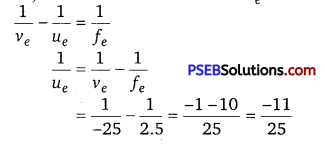∴ ue = $$-\frac{25}{11}$$ = -2.27 cm
We can also obtain the value of the image distance for the objective lens (v0) using the lens formula.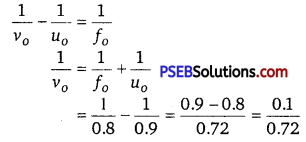∴ v0 = 7.2 cm
The distance between the objective lens and the eyepiece = |ue|+v0
= 2.27+ 7.2 = 9.47cm
The magnifying power of the microscope is calculated as $$\frac{v_{o}}{\left|u_{o}\right|}\left(1+\frac{d}{f_{e}}\right)$$
= $$\frac{7.2}{0.9}\left(1+\frac{25}{2.5}\right)$$
= 8(1 +10) = 88
Hence, the magnifying power of the microscope is 88.

Question 13.
A small telescope has an objective lens of focal length 144 cm and an eyepiece of focal length 6.0cm. What is the magnifying power of the telescope? What is the separation between the objective and the eyepiece?
Focal length of the objective lens, f0 = 144 cm
Focal length of the eyepiece, fe = 6.0 cm
The magnifying power of the telescope is given as, m = $$\frac{f_{o}}{f_{e}}=\frac{144}{6}$$ = 24
The separation between the objective lens and the eyepiece is calculated as
= fo + fe
= 144 + 6 = 150 cm
Hence, the magnifying power of the telescope is 24 and the separation between the objective lens and the eyepiece is 150 cm.

Question 14.
(a) A giant refracting telescope at an observatory has an objective lens of focal length 15 m. If an eyepiece of focal length 1.0 cm is used, what is the angular magnification of the telescope?
(b) If this telescope is used to view the moon, what is the diameter of the image of the moon formed by the objective lens? The diameter of the moon is 3.48 x 106 m, and the radius of lunar orbit is 3.8 x 108 m.
(a) Given f0 = 15 m,
fe = 1.0 cm = 1.0 x 10-2 m
Angular magnification of telescope,
m = $$-\frac{f_{o}}{f_{e}}=-\frac{15}{1.0 \times 10^{-2}}$$ = -1500
Negative sign shows that the final image is inverted.
(b) Let D be diameter of moon, d diameter of image of moon formed by objective and r be the distance of moon from objective lens, then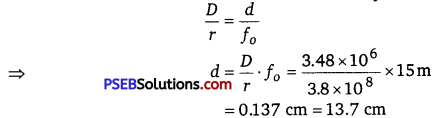Question 15.
Use the mirror equation to deduce that:
(a) an object placed between f and 2f of a concave mirror produces a real image beyond 2f.
(b) a convex mirror always produces a virtual image independent of the location of the object.
(c) the virtual image produced by a convex mirror is always diminished In size and is located between the focus and the
pole.
(d) an object placed between the pole and focus of a concave mirror produces a virtual and enlarged image.
[Note: This exercise helps you deduce algebraically properties of images that one obtains from explicit ray diagrams.]
(a) For a concave mirror, the focal length (f) is negative
∴ f<o
When the object is placed on the left side of the mirror, the object distance (u) is negative.
∴ u<O
For image distance v, we can write the mirror formula
$$\frac{1}{v}=\frac{1}{f}-\frac{1}{u}$$ …………………………………… (1)
The object lies between f and 2f.
∴ 2f < u < f (∵ u and f are negative) ∴ $$\frac{1}{2 f}>\frac{1}{u}>\frac{1}{f}$$
$$-\frac{1}{2 f}<-\frac{1}{u}<-\frac{1}{f}$$
$$\frac{1}{f}-\frac{1}{2 f}<\frac{1}{f}-\frac{1}{u}<0$$ ………………………………… (2)
Using equation (1), we get
$$\frac{1}{2 f}<\frac{1}{v}<0$$

∴ $$\frac{1}{v}$$ is negative, i.e., v is negative.
$$\frac{1}{2 f}<\frac{1}{v}$$ 2f > v
-v > -2 f
Therefore, the image lies beyond 2f.

(b) For a convex mirror, the focal length (f) is positive.
∴ f>o
When the object is placed on the left side of the mirror, the object distance (u) is negative.
∴ u<O
For image distance y, we have the mirror formula
$$\frac{1}{v}=\frac{1}{f}-\frac{1}{u}$$
Using equation (2), we can conclude that
$$\frac{1}{\nu}$$ < 0 v v> 0
Thus, the image is formed on the back side of the mirror.
Hence, a convex mirror always produces a virtual image, regardless of the object distance.

(c) For a convex mirror, the focal length (f) is positive.
∴ f> 0
When the object is placed on the left side of the mirror, the object distance (u) is negative.
∴ u< 0
For image distance v, we have the mirror formula
$$\frac{1}{v}=\frac{1}{f}-\frac{1}{u}$$
But we have u < 0 ∴ $$\frac{1}{v}>\frac{1}{f}$$
v < f
Hence, the image formed is diminished and is located between the focus (f) and the pole.

(d) For a concave mirror, the focal length (f) is negative.
∴ f< 0
When the object is placed on the left side of the mirror, the object distance (u) is negative.
∴ u< 0 It is placed between the focus (f) and the pole. ∴f > u > 0
$$\frac{1}{f}<\frac{1}{u}$$ < 0 $$\frac{1}{f}-\frac{1}{u}$$ > 0
For image distance v, we have the mirror formula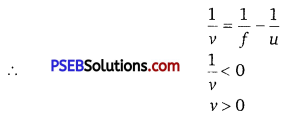The image is formed on the right side of the mirror. Hence, it is a virtual image.
For u < 0 and v > 0, we can write
$$\frac{1}{u}>\frac{1}{v}$$
v > u
Magnification, m = $$\frac{v}{u}$$ > 1 u
Hence, the formed image is enlarged.

Question 16.
A small pin fixed on a tabletop is viewed from above from a distance of 50 cm. By what distance would the pin appear to be raised if it is viewed from the same point through a 15 cm thick glass slab held parallel to the table? Refractive index of glass = 1.5. Does the answer depend on the location of the slab?
Actual depth of the pin, d = 15cm
Apparent depth of the pin = d’
Refractive index of glass, µ = 1.5

Ratio of actual depth to the apparent depth is equal to the refractive index of glass, i.e.
µ = $$\frac{d}{d^{\prime}}$$
∴ d’ = $$\frac{d}{\mu}$$
= $$\frac{15}{1.5}$$ = 10 cm
The distance at which the pin appears to be raised = d-d’=15-10 = 5 cm
For a small angle of incidence, this distance does not depend upon the location of the slab.

Question 17.
(a) Figure 9.35 shows a cross-section of a ‘light pipe’ made of a glass fibre of refractive index 1.68. The outer covering of the pipe is made of a material of refractive index 1.44. What is the range of the angles of the incident rays with the axis of the pipe for which total reflections inside the pipe take place, as shown in the figure.
(b) What is the answer if there is no outer covering of the pipe?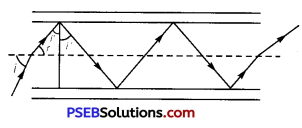(a) Refractive index of the glass fibre, µ2 = 1.68
Refractive index of the outer covering of the pipe, µ1 =1.44
Angle of incidence = i
Angle of refraction = r
Angle of incidence at the interface = i’

The refractive index (µ) of the inner core-outer core interface is given as
µ = $$\frac{\mu_{2}}{\mu_{1}}=\frac{1}{\sin i^{\prime}}$$
sin i’ = $$\frac{\mu_{1}}{\mu_{2}}=\frac{1.44}{1.68}$$ = 0.8571
∴ i’ = 59°

For the critical angle, total internal reflection (TIR) takes place only when i > i’. i.e., i > 59°
Maximum angle of reflection, rmax = 90°-i’ = 90°-59°= 31°
Let, imax be the maximum angle of incidence.
The refractive index at the air – glass interface, µ2 =1.68
µ2 = $$\frac{\sin i_{\max }}{\sin r_{\max }}$$
sin imax = µ2 sin rmax = 1.68 sin31°
= 1.68 x 0.5150
= 0.8652
∴imax = sin-1 (0.8652) ≈ 60°
Thus, all the rays incident at angles lying in the range 0 < i < 60° will suffer total internal reflection.

(b) If the outer covering of the pipe is not present, then
Refractive index of the outer pipe, µ1 = Refractive index of air = 1
For the angle of incidence i =90°, we can write Snell’s law at the air-pipe interface as
$$\frac{\sin i}{\sin r}$$ = µ2 = 1.68
sin r = $$\frac{\sin 90^{\circ}}{1.68}=\frac{1}{1.68}$$
r = sin-1(0.5952)
∴ i’ = 90°-36.5°= 53.5°
Since i’ > r, all incident rays will suffer total internal reflection.

Question 18.
(a) You have learnt that plane and convex mirrors produce virtual images of objects. Can they produce real images under some circumstances? Explain.
(b) A virtual image, we always say, cannot be caught on a screen. Yet when we ‘see’ a virtual image, we are obviously bringing it onto the ‘screen’(i.e., the retina) of our eye. Is there a contradiction?
(c) A diver underwater, looks obliquely at a fisherman standing on the bank of a lake. Would the fisherman look taller or shorter to the diver than what he actually is?
(d) Does the apparent depth of a tank of water change if viewed obliquely? If so, does the apparent depth increase or decrease?
(e) The refractive index of diamond is much greater than that – of ordinary glass. Is this fact of some use to a diamond cutter?
(a) Yes, they produce real images under some circumstances. If the object is virtual, i.e., if the light rays converging at a point behind a plane mirror (or a convex mirror) are reflected to a point on a screen placed in front of the mirror, then a real image will be formed.

(b) No, there is no contradiction. A virtual image is formed when light rays diverge. The convex lens of the eye causes these divergent rays to converge at the retina. In this case, the virtual image serves as an object for the lens to produce a real image.

(c) The diver is in the water and the fisherman is on land (i.e., in the air). Water is a denser medium than air. It is given that the diver is viewing the fisherman. This indicates that the light rays are traveling from a denser medium to a rarer medium. Hence, the refracted rays will move away from the normal. As a result, the fisherman will appear to be taller.

(d) Yes, the apparent depth of a tank of water changes when viewed obliquely. This is because light bends on traveling from one medium to another. The apparent depth of the tank, when viewed obliquely, is less than the near-normal viewing.

(e) Yes, the refractive index of diamond (2.42) is more than that of ordinary glass (1.5). The critical angle for diamond is less than that for glass. A diamond cutter uses a large angle of incidence to ensure that the light entering the diamond is totally reflected from its faces. This is the reason for the sparkling effect of a diamond.

Question 19.
The image of a small electric bulb on the wall of a room is to be obtained on the opposite wall 3 m away by means of a large convex lens. What is the maximum possible focal length of the lens required for the purpose? ’’
Here, u + v = 3 m, :.v = 3 -u
From lens formula,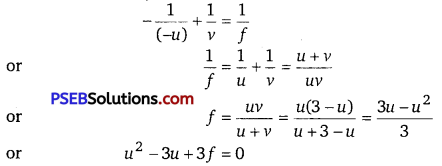or u = $$\frac{3 \pm \sqrt{3^{2}-4.3 f}}{2}$$
For real solution, 9 -12, f should be positive.
It., 9 -12f > 0
or 9 >12f.
or f < $$\frac{9}{12}$$ < $$\frac{3}{4}$$ m
∴ The maximum focal length of the lens required for the purpose is $$\frac{3}{4}$$ m
i.e, fmax = 0.7 m

Question 20.
A screen is placed 90 cm from an object. The image of the object on the screen is formed by a convex lens at two different locations separated by 20 cm. Determine the focal length of the lens.
Here, O is a position of object and I is position of image (screen).
Distance OI = 90 cm
L1 and L2 are the two positions of the lens.
∴ Distance between L1 and L2 = O1 O2 = 20 cm
For Position L1 of the Lens: Let x be the distance of the object from the lens.
∴ u1 = -x
∴ Distance of the image from the lens, v1 = +(90 – x)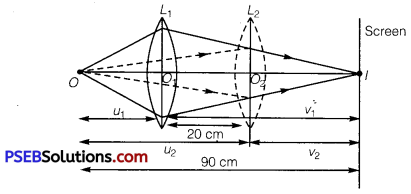If f be the focal length of the lens, then using lens formula,
$$-\frac{1}{u}+\frac{1}{v}=\frac{1}{f}$$ we get
$$-\frac{1}{-x}+\frac{1}{90-x}=\frac{1}{f}$$
or $$\frac{1}{f}=\frac{1}{x}+\frac{1}{90-x}$$ ……………………………….. (1)
For Position L2 of the Lens : Let u2 and v2 be the distances of the object and image from the lens in this position.
∴ u2=-(X + 20),
v2 = +[90-(x+20)] = +(70-x)
∴ Using lens formula,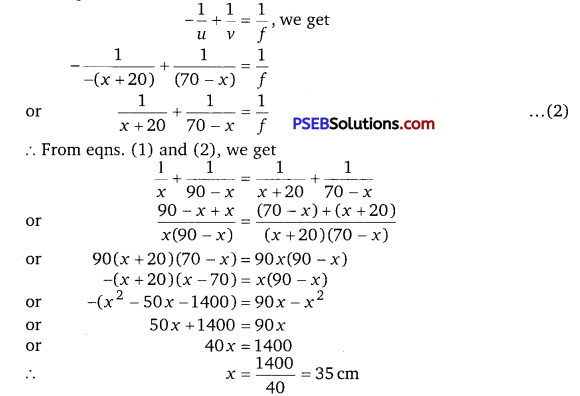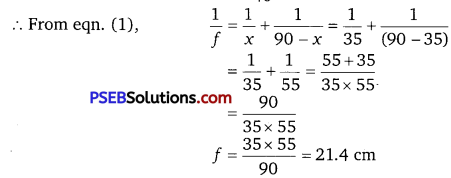Question 21.
(a) Determine the ‘effective focal length’ of the combination of the two lenses in Exercise 9.10, if they are placed 8.0 cm apart with their principal axes coincident. Does the answer depend on which side of the combination a beam of parallel light is incident? Is the notion of effective focal length of this system useful at all?

(b) An object 1.5 cm in size is placed on the side of the convex lens in the arrangement (a) above. The distance between the object and the convex lens is 40 cm. Determine the magnification produced by the two-lens system and the size of the image.
Focal length of the convex lens, f1 = 30 cm
Focal length of the concave lens,f2 = -20 cm
Distance between the two lenses, d = 8.0 cm

(a)
(i) When the parallel beam of light is incident on the convex lens first.
According to the lens formula, we have
$$\frac{1}{v_{1}}-\frac{1}{u_{1}}=\frac{1}{f_{1}}$$
where, μ1 = Object distance = ∞, v1 = Image distance = ?
$$\frac{1}{v_{1}}=\frac{1}{30}-\frac{1}{\infty}=\frac{1}{30}$$
∴ v1 = 30 cm
The image will act as a virtual object for the concave lens. Applying lens formula to the concave lens, we have
$$\frac{1}{v_{2}}-\frac{1}{u_{2}}=\frac{1}{f_{2}}$$
where, u2 = Object distance = (30 – d) = 30 – 8 = 22 cm,
v2 = Image distance=?
$$\frac{1}{v_{2}}=\frac{1}{22}-\frac{1}{20}=\frac{10-11}{220}=\frac{-1}{220}$$
∴ v2 = -220 cm
The parallel incident beam appears to diverge from a point that is $$\left(220-\frac{d}{2}=220-\frac{8}{2}=220-4=216 \mathrm{~cm}\right)$$ from the centre of the combination of the two lenses.

(ii) When the parallel beam of light is incident, on the concave lens first. According to the lens formula, we have
$$\frac{1}{v_{2}}-\frac{1}{u_{2}}=\frac{1}{f_{2}}$$
$$\frac{1}{v_{2}}=\frac{1}{f_{2}}+\frac{1}{u_{2}}$$
where, u2 = Object distance = -∞, v2 = Image distance = ?
$$\frac{1}{v_{2}}=\frac{1}{-20}+\frac{1}{-\infty}=-\frac{1}{20}$$
∴ v2 = -20 cm
The image will act as a real object for the .convex lens.
Applying lens formula to the convex lens, we have
$$\frac{1}{v_{1}}-\frac{1}{u_{1}}=\frac{1}{f_{1}}$$
where, u1 = Object distance = -(20 + d) = -(20 + 8) = -28 cm v1 = Image distance = ?
$$\frac{1}{v_{1}}=\frac{1}{30}+\frac{1}{-28}=\frac{14-15}{420}=\frac{-1}{420}$$
∴ v1 = -420 cm
Hence, the parallel incident beam appear to diverge from a point that is (420 – 4 = 416 cm) from the left of the centre of the combination of the two lenses. The answer depends on the side of the combination at which the parallel beam of light is incident. The notion of effective focal length does not seem to be useful for this combination.

(b) Height of the object, h1 =1.5 cm
Object distance from the side of the convex lens, u1 = -40 cm
|ui| = 40 cm

According to the lens formula
$$\frac{1}{v_{1}}-\frac{1}{u_{1}}=\frac{1}{f_{1}}$$
where, v1 = Image distance =?
$$\frac{1}{v_{1}}=\frac{1}{30}+\frac{1}{-40}=\frac{4-3}{120}=\frac{1}{120}$$
∴ v1 = 120 cm
Magnification, m= $$\frac{v_{1}}{\left|u_{1}\right|}=\frac{120}{40}$$ = 3

Hence, the magnification due to the convex lens is 3.
The image formed by the convex lens acts as an object for the concave lens.
According to the lens formula
$$\frac{1}{v_{2}}-\frac{1}{u_{2}}=\frac{1}{f_{2}}$$
where,
u2 = Object distance = +(120 —8)=112 cm
v2= Image distance =?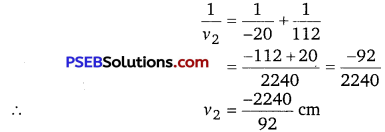Magnification, m’ = $$\left|\frac{v_{2}}{u_{2}}\right|=\frac{2240}{92} \times \frac{1}{112}=\frac{20}{92}$$
Hence, the magnification due to the concave lens is $$\frac{20}{92}$$
The magnification produced by the combination of the two lenses is calculated as m x m’ = $$3 \times \frac{20}{92}=\frac{60}{92}$$ = 0.652
The magnification of the combination is given as
$$\frac{h_{2}}{h_{1}}$$ = 0.652
h2 = 0.652 x h1
where, h1 = Object size = 1.5 cm,
h2 = Size of the image
∴ h2 = 0.652 x 1.5 = 0.98 cm
Hence, the height of the image is 0.98 cm.

Question 22.
At what angle should a ray of light be incident on the face of a prism of refracting angle 60° so that it just suffers total internal reflection at the other face? The refractive index of the material of the prism is 1.524.
The incident, refracted, and emergent rays associated with a glass prism ABC are shown in the given figure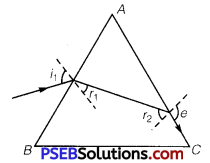Angle of prism, A = 60°
Refractive index of the prism, μ = 1.524
i1 = Incident angle
r2 = Refracted angle
r2 = Angle of incidence at the face
AC = e = Emergent angle = 90°

According to Snell’s law, for face AC, we can have sine
$$\frac{\sin e}{\sin r_{2}}$$ = μ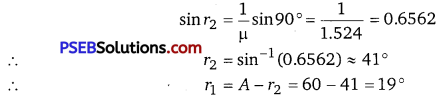It is clear from the figure that angle A = r1 + r2
According to Snell’s law, we have the relation
μ = $$\frac{\sin i_{1}}{\sin r_{1}}$$
sin i1 = μ sin r1
= 1.524 x sin19°= 0.496
∴ i1= 29.75°
Hence, the angle of incidence is 29.75°.

Question 23.
You are given prisms made of crown glass and flint glass with a wide variety of angles. Suggest a combination of prisms which will
(a) deviate a pencil of white light without much dispersion,
(b) disperse (and displace) a pencil of white light without much deviation.
(a) Place the two prisms beside each other. Make sure that their bases are on the opposite sides of the incident white light, with their faces touching each other. When the white light is incident on the first prism, it will get dispersed. When this dispersed light is incident on the second prism, it will recombine and white light will emerge from the combination of the two prisms.

(b) Take the system of the two prisms as suggested in answer (a). Adjust (increase) the angle of the flint-glass-prism so that the deviations due to the combination of the prisms become equal. This combination will disperse the pencil of white light without much deviation.

Question 24.
For a normal eye, the far point is at infinity and the near point of distinct vision is about 25 cm in front of the eye. The cornea of . the eye provides a converging power of about 40 dioptres, and the least converging power of the eye-lens behind the cornea is about 20 dioptres. From this rough data estimate the range of accommodation (i.e., the range of converging power of the eye-lens) of a normal eye.
Least distance of distinct vision, d = 25 cm
Far point of a normal eye, d’ = ∞
Converging power of the cornea, Pc = 40 D
Least converging power of the eye- lens, Pe = 20 D
To see the objects at infinity, the eye uses its least converging power.
Power of the eye-lens, P = Pc + Pe =40+20 = 60 D
Power of the eye-lens is given as
P = $$\frac{1}{\text { Focal length of the eye lens }(f)}$$
f = $$=\frac{1}{P}=\frac{1}{60 D}=\frac{100}{60}=\frac{5}{3}$$ cm

To focus an object at the near point, object distance (u) = -d = -25 cm
Focal length of the eye-lens = Distance between the cornea and the retina = Image distance
Hence, image distance, v = $$\frac{5}{3}$$ cm
According to the lens formula, we can write
$$\frac{1}{f^{\prime}}=\frac{1}{v}-\frac{1}{u}$$
Where f’ = Focal length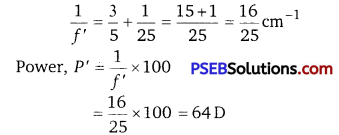Power of the eye-lens = 64-40 = 24 D
Hence, the range of accommodation of the eye-lens is from 20 D to24D.

Question 25.
Does short-sightedness (myopia) or long-sightedness (hypermetropia) imply necessarily that the eye has partially lost its ability of accommodation? If not, what might cause these defects of vision?
A myopic or hypermetropic person can also possess the normal ability of accommodation of the eye-lens. Myopia occurs when the eyeballs get elongated from front to back. Hypermetropia occurs when the eye-balls get shortened.
When the eye-lens loses its ability of accommodation, the defect is called presbyopia.

Question 26.
A myopic person has been using spectacles of power -1.0 dioptre for distant vision. During old age he also needs to use separate reading glass of power +2.0 dioptres. Explain what may have happened.
The power of the spectacles used by the myopic person, P = -1.0 D
Focal length of the spectacles, f = $$\frac{1}{P}=\frac{1}{-1 \times 10^{-2}}$$ = -100 cm
Hence, the far point of the person is 100 cm. He might have a normal near point of 25 cm. When he uses the spectacles, the objects placed at infinity produce virtual images at 100 cm.
He uses the ability of accommodation of the eye-lens to see the objects placed between 100 cm and 25 cm.
During old age, the person uses reading glasses of power, P’ = +2D The ability of accommodation is lost in old age.
This defect is called presbyopia. As a result, he is unable to see clearly the objects placed at 25 cm.

Question 27.
A person looking at a person wearing a shirt with a pattern comprising vertical and horizontal lines is able to see the vertical lines more distinctly than the horizontal ones. What is this defect due to? How is such a defect of vision corrected?
In the given case, the person is able to see vertical lines more distinctly than horizontal lines. This means that the refracting system (cornea and eye-lens) of the eye is not working in the same way in different planes. This defect is tailed astigmatism. The person’s eye has enough curvature in the vertical plane. However, the curvature in the horizontal plane is insufficient. Hence, sharp images of the vertical lines are formed on the retina, but horizontal lines appear blurred. This defect can be corrected by using cylindrical lenses.

Question 28.
A man with normal near point (25cm) reads a book with small print using a magnifying glass: a thin convex lens of focal length 5 cm.
(a) What is the closest and the farthest distance at which he should keep the lens from the page so that he can read the book when viewing through the magnifying glass?
(b) What is the maximum and the minimum angular magnification (magnifying power) possible using the above simple microscope?
(a) Focal length of the magnifying glass, f = 5 cm
Least distance of distinct vision, d = 25 cm
Closest object distance = u
Image distance, v = -d = -25 cm
According to the lens formula, we have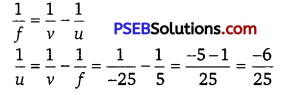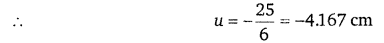Hence, the closest distance at which the person can read the book is 4.167 cm.
For the object at the farthest distance (u’), the image distance (v’) = ∞

According to the lens formula, we have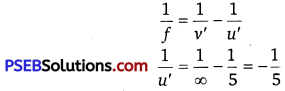∴ u’ = -5 cm
Hence, the farthest distance at which the person can read the book is 5 cm.
(b) Maximum angular magnification is given by the relation
αmax= $$\frac{d}{|u|}=\frac{25}{\frac{25}{6}}$$ = 6
Minimum angular magnification is given by the relation
αmin = $$\frac{d}{\left|u^{\prime}\right|}=\frac{25}{5}$$ = 5.

Question 29.
A card sheet divided into squares each of size 1 mm2 is being viewed at a distance of 9 cm through a magnifying glass (a converging lens of focal length 10 cm) held close to the eye.
(a) What is the magnification produced by the lens? How much is the area of each square in the virtual image?
(b) What is the angular magnification (magnifying power) of the lens?
(c) Is the magnification in (a) equal to the magnifying power in (b)?
Explain.
(a) Area of each square, A = 1 mm2
Object distance, u = -9 cm
Focal length of the converging lens, f = 10 cm
For image distance v, the lens formula can be written as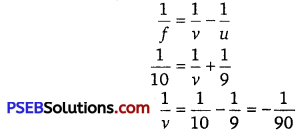∴ v = -90 cm
Magnification, m = $$=\frac{v}{u}=\frac{-90}{-9}$$ =10
∴ Area of each square in the virtual image = (10)2A
= 102 x 1 =100 mm2 = 1 cm2
(b) Magnifying power of the lens = $$\frac{d}{|u|}=\frac{25}{9}$$ = 2.8
(c) The magnification in (a) is not the same as the magnifying power in(b).
The magnification magnitude is $$\left(\left|\frac{v}{u}\right|\right)$$ and the magnifying power is $$\left(\frac{d}{|u|}\right)$$ .
The two quantities will be equal when the image is formed at the near point (25 cm).

Question 30.
(a) At what distance should the lens be held from the figure in Exercise 9.29 in order to view the squares distinctly with the maximum possible magnifying power?
(b) What is the magnification in this case?
(c) Is the magnification equal to the magnifying power in this case? Explain.
(a) The maximum possible magnification is obtained when the image is formed at the near point (d = 25cm).
Image distance, v = -d = -25 cm
Focal length, f = 10 cm
Object distance = u
According to the lens formula, we have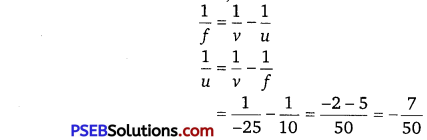∴ u = $$-\frac{50}{7}$$ = -7.14 cm
Hence, to view the squares distinctly, the, lens should be kept 7.14 cm away from them. .
(b) Magnifying = $$\left|\frac{v}{u}\right|=\frac{25}{50}$$ =3.5
(c) Magnifying power = $$\frac{d}{u}=\frac{25}{\frac{50}{7}}$$ = 3.5
Since the image is formed at the near point (25 cm), the magnifying power is equal to the magnitude of magnification.

Question 31.
What should be the distance between the object in Exercise 9.30 and the magnifying glass if the virtual image of each square in the figure is to have an area of 6.25 mm2. Would you be able to see the squares distinctly with your eyes very close to the magnifier? [Note: Exercises 9.29 to 9.31 will help you clearly understand the difference between magnification in absolute size and the angular magnification (or magnifying power) of an instrument.]
Area of the virtual image of each square, A = 6.25 mm
Area of each square, A0 = 1 mm2
Hence, the linear magnification of the object can be calculated as
m = $$\sqrt{\frac{A}{A_{0}}}=\sqrt{\frac{6.25}{1}}$$ = 2.5
But m = $$\frac{\text { Image distance }(v)}{\text { Object distance }(u)}$$
∴ v = mu = 2.5 u
Focal length of the magnifying glass, f = 10 cm

According to the lens formula, we have the relation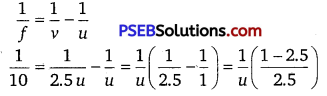∴ u = $$-\frac{1.5 \times 10}{2.5}$$ = -6 cm
and v = 2.5 u = 2.5 x 6 = -15 cm
The virtual image is formed at a distance of 15 cm, which is less than the near point (i.e., 25 cm) of a normal eye. Hence, it cannot be seen by the eyes distinctly.

Question 32.
(a) The angle subtended at the eye by an object is equal to the angle subtended at the eye by the virtual image produced by a magnifying glass. In what sense then does a magnifying glass provide angular magnification?
(b) In viewing through a magnifying glass, one usually positions one’s eyes very close to the lens. Does angular magnification change if the eye is moved back?
(c) Magnifying power of a simple microscope is inversely proportional to the focal length of the lens. What then stops us from using a convex lens of smaller and smaller focal length and achieving greater and greater magnifying power?
(d) Why must both the objective and the eyepiece of a compound microscope have short focal lengths?
(e) When viewing through a compound microscope, our eyes should be positioned not on the eyepiece but a short distance away from it for best viewing. Why? How much should be that short distance between the eye and eyepiece?
(a) Though the image size is bigger than the object, the angular size of the image is equal to the angular size of the object. A magnifying glass helps one see the objects placed closer than the least distance of distinct vision (i.e., 25 cm). A closer object causes a larger angular size. A magnifying glass provides angular magnification. Without magnification, the object cannot be placed closer to the eye. With magnification, the object can be placed much closer to the eye.

(b) Yes, the angular magnification changes when the distance between the eye and a magnifying glass is increased, the angular magnification decreases a little. This is because the angle subtended at the eye is slightly less than the angle subtended at the lens. Image distance does not have any effect on angular magnification.

(c) The focal length of a convex lens cannot be decreased by a greater amount. This is because making lenses having very small focal lengths is not easy. Spherical and chromatic aberrations are produced by a convex lens having a very small focal length.

(d) The ang lar magificarin produced by’the eyepiece of a compound microscope is $$\left[\left(\frac{25}{f_{e}}\right)+1\right]$$
Where fe = Focal length of the eyepiece
It can be inferred that fe is small, then angular magnification of the eyepiece will be large.
The angular magnification of the objective lens of a compound microscope is given as
$$\frac{1}{\left(\left|u_{o}\right| f_{o}\right)}$$
Where, u0 = Object distance for the objective lens, f0 = Focal length of the objective
The magnification is large when u0> f0 . In the case of a microscope, the object is kept close to the objective lens. Hence, the object distance is very little.
Since u0 is small, f0 will be even smaller. Therefore, fe and f0 are both small in the given condition.

(e) When we place our eyes too close to the eyepiece of a compound microscope, we are unable to collect much-refracted light. As a result, the field of view decreases substantially. Hence, the clarity of the image gets blurred.
The best position of the eye for viewing through a compound microscope is at the eye-ring attached to the eyepiece. The precise location of the eye depends on the separation between the objective lens and the eyepiece.

Question 33.
An angular magnification (magnifying power) of 30X is desired using an objective of focal length 1.25 cm and an eyepiece of focal length 5 cm. How will you set up the compound microscope?
Focal length of the objective lens, f0 = 1.25 cm
Focal length of the eyepiece, fe = 5 cm
Least distance of distinct vision, d = 25 cm

Angular magnification of the compound microscope = 30X
Total magnifying power of the compound microscope, m = 30
The angular magnification of the eyepiece is given by the relation
me = $$\left(1+\frac{d}{f_{e}}\right)=\left(1+\frac{25}{5}\right)$$ = 1+5 = 6
The angular magnification of the objective lens (m0) is related to me as
mome=m
or m0 = $$\frac{m}{m_{e}}=\frac{30}{6}$$ = 5

We also have the relation
m = $$\frac{\text { Image distance for the objective lens }\left(v_{o}\right)}{\text { Object distance for the objective lens }\left(-u_{0}\right)}$$
5 = $$\frac{v_{o}}{-u_{o}}$$
∴ v0 = -5u0 …………………………….. (1)
Applying the lens formula for the objective lens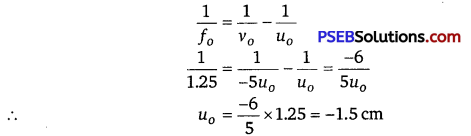and v0 = -5u0
= -5 x (-1.5) = 7.5 cm
The object should be placed 1.5 cm away from the objective lens to obtain the desired magnification.
Applying the lens formula for the eyepiece
$$\frac{1}{v_{e}}-\frac{1}{u_{e}}=\frac{1}{f_{e}}$$
where,
ve = Image distance for the eyepiece = -d = -25 cm
ue = Object distance for the eyepiece
$$\frac{1}{u_{e}}=\frac{1}{v_{e}}-\frac{1}{f_{e}}=\frac{-1}{25}-\frac{1}{5}=-\frac{6}{25}$$
ue =-4.17 cm
Separation between the objective lens and the eyepiece = $$\left|u_{e}\right|+\left|v_{o}\right|$$
= 4.17 + 7.5 = 11.67 cm
Therefore, the separation between the objective lens and the eyepiece should be 11.67 cm.

Question 34.
A small telescope has an objective lens of focal length 140 cm and an eyepiece of focal length 5.0 cm. What is the magnifying power of the telescope for viewing distant objects when
(a) the telescope is in normal adjustment (i.e., when the final image is at infinity)?
(b) the final image is formed at the least distance of distinct vision (25 cm)?
Focal length of the objective lens, f0 =140 cm
Focal length of the eyepiece, fe = 5 cm
Least distance of distinct vision, d = 25 cm
(a) When the telescope is in normal adjustment, its magnifying power is given as
m = $$\frac{f_{o}}{f_{e}}=\frac{140}{5}$$ = 28
(b) When the final image is formed at d, the magnifying power of the telescope is given as
$$\frac{f_{o}}{f_{e}}\left[1+\frac{f_{e}}{d}\right]=\frac{140}{5}\left[1+\frac{5}{25}\right]$$
= 28[1 +0.2] = 28×1.2 = 33.6

Question 35.
(a) For the telescope described in Exercise 9.34 (a), what is the separation between the objective lens and the eyepiece?
(b) If this telescope is used to view a 100 m tall tower 3 km away, what is the height of the image of the tower formed by the objective lens?
(c) What is the height of the final image of the tower if it is formed at 25 cm?
Focal length of the objective lens, f0 =140 cm
Focal length of the eyepiece, fe= 5 cm
(a) In normal adjustment, the separation between the objective lens and the eyepiece = f0 + fe = 140 + 5 = 145 cm
(b) Height of the tower, h1 = 100 m
Distance of the tower (object) from the telescope, u = 3 km = 3000 m
The angle subtended by the tower at the telescope is given as
θ’ = $$\frac{h_{2}}{f_{o}}=\frac{h_{2}}{140}$$ rad
where,
h2 = Height of the image of the tower formed by the objective lens
$$\frac{1}{30}=\frac{h_{2}}{140}$$ (∵θ=θ’)
∴ h2 = $$\frac{140}{30}$$ = 4.7 cm
Therefore, the objective lens forms a 4.7 cm tall image of the tower.

(c) Image is formed at a distance, d = 25 cm
The magnification of the eyepiece is given by the relation
m = 1 + $$\frac{d}{f_{e}}$$
= 1+ $$\frac{25}{5}$$ =1 + 5 = 6
Height of the final image = mh2 = 6 x 4.7 = 28.2 cm
Hence, the height of the final image of the tower is 28.2 cm.

Question 36.
A Cassegrain telescope uses two mirrors as shown in Fig. 9.33. Such a telescope is built with the mirrors 20 mm apart.
If the radius of curvature of the large mirror is 220 mm and the small mirror is 140 mm, where will the final image of an object at infinity be?
Given, r1 = 220 mm, f1 = $$\frac{r_{1}}{2}$$ = 110 mm = 11 cm
r2 = 140 mm, f2 = $$\frac{r_{2}}{2}$$ = 70 mm = 7.0 cm
Distance between mirrors, d = 20 mm = 2.0 cm
The parallel incident rays coming from distant objects fall on the concave mirror and try to be focused at the principal focus of concave lens, i. e., v1 = -f1 = -11 cm
But in the path of rays reflected from concave mirror, a convex mirror is placed. Therefore the image formed by the concave mirror acts as a virtual object for convex mirror.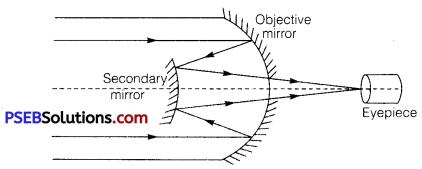For convex mirror f2 = -7.0 cm, u2 = -(11 -2) = -9 cm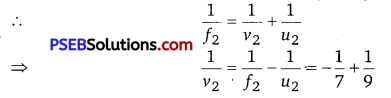v2 = $$-\frac{63}{2}$$ cm = -31.5 cm
This is the distance of the final image formed by the convex mirror. Thus, the final image is formed at a distance of 31.5 cm from the smaller (convex) mirror behind the bigger mirror.

Question 37.
Light incident normally on a plane mirror attached to a galvanometer coil retraces backward as shown in Fig. 9.36. A current in the coil produces a deflection of 3.5° of the mirror. What is the displacement of the reflected spot of light on a screen placed 1.5 m away?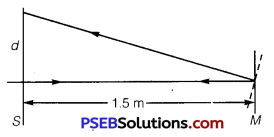Angle of deflection, θ = 3.5°
Distance of the screen from the mirror, D = 1.5 m
The reflected rays get deflected by an amount twice the angle of deflection i.e., 2θ = 2 x 3.5 = 7.0°
The displacement (d) of the reflected spot of light on the screen is given as
tan 2θ = $$\frac{d}{1.5}$$ d =1.5 x tan7°= 0.184 m = 18.4 cm
Hence, the displacement of the reflected spot of light is 18.4 cm.

Question 38.
Figure 9.37 shows an biconvex lens (of refractive index 1.50) in contact with a liquid layer on top of a plane mirror.
A small needle with its tip on the principal axis is moved along the axis until its inverted image is found at the position of the needle. The distance of the needle from the lens is measured to be 45.0 cm. The liquid is removed and the experiment is repeated. The new distance is measured to he 30.0 cm. What is the refractive index of the liquid?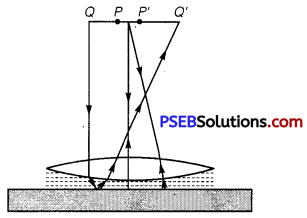Focal length of the convex lens, f1 = 30 cm
The liquid acts as a mirror. Focal length of the liquid = f2
Focal length of the system (convex lens + liquid), f = 45 cm
For a pair of optical systems placed in contact, the equivalent focal length is given as
$$\frac{1}{f}=\frac{1}{f_{1}}+\frac{1}{f_{2}}$$
$$\frac{1}{f_{2}}=\frac{1}{f}-\frac{1}{f_{1}}$$
= $$\frac{1}{45}-\frac{1}{30}=-\frac{1}{90}$$
∴ f2 = -90 cm
Let the refractive index of the lens be μ1 and the radius of curvature of one surface be R. Hence, the radius of curvature of the other surface is R.
R can be obtained using the relation $$\frac{1}{f_{1}}=\left(\mu_{1}-1\right)\left(\frac{1}{R}+\frac{1}{-R}\right)$$
$$\frac{1}{30}=(1.5-1)\left(\frac{2}{R}\right)$$
∴ R = $$\frac{30}{0.5 \times 2}$$ = 30 cm

Let μ2 be the refractive index of the liquid.
Radius of curvature of the liquid on the side of the plane minor = ∞
Radius of curvature of the liquid on the side of the lens, R = -30 cm
The value of μ2, can be calculated using the relation
$$\frac{1}{f_{2}}=\left(\mu_{2}-1\right)\left[\frac{1}{-R}-\frac{1}{\infty}\right]$$
$$\frac{-1}{90}=\left(\mu_{2}-1\right)\left[\frac{1}{+30}-0\right]$$
μ2 – 1 = $$\frac{1}{3}$$
∴ μ2 = $$\frac{4}{3}$$ = 133
Hence, the refractive index of the liquid is 1.33.